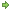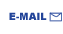Home Fractals Tutorials Books Archive My blog My LinkedIn Profile Follow @lano1106BOOKS i'm reading```/*
* Name   : graph.cpp
* Purpose: Find the shortest distance between 2 vertices in a graph
*          by using graph breadth-first traversal
*          This program has been developped as an assignment during a remote interview process
*
* Known limitation: Another approach would be required on a weighted graph
*                   such computing the minimum span tree of the graph.
*
* I recommend Robert Sedgewick excellent book on algorithms
*
* Author : Olivier Langlois <olivier@olivierlanglois.net>
*
* Date: October 05, 2009
*/

#include <ctype.h>    // For isalpha(), islower()
#include <set>
#include <deque>
#include <bitset>
#include <vector>
#include <iostream>
#include <sstream>    // For std::ostringstream
#include <iterator>   // For std::ostream_iterator and std::iterator
#include <algorithm>  // For std::copy(), count_if() and for_each()
#include <stdexcept>  // For std::runtime_error
#include <functional> // For std::binary_function, bind2nd and not_equal_to

namespace {

const unsigned MAXNODES = 52;

// forward declaration
class Node;

/*
* Predicate to sort Node pointers by ascending order of their ids.
*/
class NodePtrPred : public std::binary_function<Node*,Node*,bool>
{
public:
bool operator()( const Node *a, const Node *b ) const;
};

/*
* class Node
*
* Purpose: Contains a reference to all neighbors nodes.
*          Note that if the graph is undirected, all neighbors will
*          also point to this Node.
*/
class Node
{
public:
typedef std::set<Node *,NodePtrPred> NeighborsCont_t;

Node(unsigned id);

static unsigned index( char a );
static char     name( unsigned idx );

// getters
unsigned getId()   const { return m_id; }
char     getName() const { return name(getId()); }
const NeighborsCont_t &getNeighbors() const { return m_neighbors; }

bool operator<( const Node &a ) const { return getId()<a.getId(); }
friend std::ostream &operator<<( std::ostream &o, const Node &n );

private:
unsigned            m_id; // Unique node id.
NeighborsCont_t m_neighbors;
};

/*
* Predicate that sorts Node pointers based on the class operator<
*/
bool NodePtrPred::operator()( const Node *a, const Node *b ) const
{
return *a<*b;
}

Node::Node(unsigned id)
: m_id(id)
{
}

inline void Node::AddNeighbor( Node *n )
{
/*
* Insertion will silently fail if n is already in the set.
*/
m_neighbors.insert(n);
}

/*
* Convert a letter to the corresponding Node index.
*/
unsigned Node::index( char a )
{
if( !isalpha(a) )
{
throw std::runtime_error("Vertex name is limited to alpha chars for now");
}
if( islower(a) )
{
return a-'a';
}
else // isupper(a)
{
return 26+a-'A';
}
}

/*
* Convert a node index back to its name.
*/
char Node::name( unsigned idx )
{
if( idx >= MAXNODES )
{
throw std::runtime_error("Vertex index is limited to MAXNODES");
}
if( idx < 26 )
{
return 'a'+idx;
}
else
{
return idx-26+'A';
}
}

/*
*
* Purpose: Allows to traverse a container of pointers and dereference each of them
*          when they are assigned to the output iterator.
*/
template<class iterator_t>
{
public:
explicit Deref_output_iterator_adapter(iterator_t &x) : m_iterator(x) {}

template<class T>
Deref_output_iterator_adapter &operator=( const T *x )
{
*m_iterator = *x; return *this;
}
Deref_output_iterator_adapter &operator*()     { return *this; }
Deref_output_iterator_adapter &operator++()    { ++m_iterator; return *this; }
Deref_output_iterator_adapter &operator++(int) { m_iterator++; return *this; }
private:
iterator_t &m_iterator;
};

/*
* operator= specialization for Node
*
* Note: The Deref_output_iterator_adapter is finally not that generic!
*/
typedef std::ostream_iterator<char> output_iterator_t;

template<>
template<>
{
*m_iterator = x->getName(); return *this;
}

/*
* output stream operator for the class Node.
*/
std::ostream &operator<<( std::ostream &o, const Node &n )
{
o << "Node " << n.getName() << " has " << n.m_neighbors.size()
<< " neighbors : ";
output_iterator_t out_it(o," ");
std::copy(n.m_neighbors.begin(),n.m_neighbors.end(),
return o;
}

/*
* class NodeContainer
*
* This is the class representing the graph.
*/
class NodeContainer
{
public:
NodeContainer();
~NodeContainer();

void AddEdge( char a, char b, bool bUndirected = true );

/*
* Input param:
*              a (char) Node name. (Valid range is a-z & A-Z).
*              idx (unsigned) Node index.
* Return value: A pointer on the requested node or NULL if not found.
*
* Note: Can throw a std::out_of_range exception if passed an invalid param
*       such as an idx greater than MAXNODES.
*       Can throw a std::runtime_error if the 'a' param is an invalid node name.
*/
Node *FindNode( char a )       { return FindNode(Node::index(a)); }
Node *FindNode( unsigned idx ) { return m_Nodes.at(idx); }

const Node *FindNode( char a ) const       { return FindNode(Node::index(a)); }
const Node *FindNode( unsigned idx ) const { return m_Nodes.at(idx); }

/*
* Purpose: Find the shortest distance from node a to node b by using the
*
* Return value: Number of hops between nodes or NOT_CONNECTED
*/
static const unsigned NOT_CONNECTED = static_cast<unsigned>(-1); // Will become the biggest unsigned value
unsigned distance( char a, char b ) const;

friend std::ostream &operator<<( std::ostream &o, const NodeContainer &c );

private:
/*
* Purpose: Check the existence of the node at index idx of m_Nodes vector
*          and create a Node if not present.
*
* Return value: Node at idx.
*
* Note: Can throw a std::out_of_range exception if idx is invalid.
*/
Node *CreateNode( unsigned idx );
std::vector<Node*> m_Nodes;
};

NodeContainer::NodeContainer()
: m_Nodes(MAXNODES)
{
}

NodeContainer::~NodeContainer()
{
for( unsigned i = 0; i < m_Nodes.size(); ++i )
{
/*
* No need to check for NULL as it is harmless to delete NULL.
*/
delete m_Nodes[i];
}
}

/*
* CreateNode()
*
* Purpose: Private method to create a node at index idx only if it does not exists yet.
*/
Node *NodeContainer::CreateNode( unsigned idx )
{
if( !m_Nodes.at(idx) )
{
m_Nodes[idx] = new Node(idx);
}
return m_Nodes[idx];
}

/*
*
* Purpose: Add an edge between node a and node b. Make the edge
*          bidirectional if bUndirected true
*/
void NodeContainer::AddEdge( char a, char b, bool bUndirected )
{
unsigned aIdx = Node::index(a);
unsigned bIdx = Node::index(b);

Node *nodeA = CreateNode(aIdx);
Node *nodeB = CreateNode(bIdx);

if( bUndirected )
{
}
}

/*
* distance()
*
* Purpose: Find the shortest distance from node a to node b by using the
*
* Return value: Number of hops between nodes or NOT_CONNECTED
*
* I recommend Robert Sedgewick excellent book on algorithms
*/
unsigned NodeContainer::distance( char a, char b ) const
{
// Input validation
const Node *nodeA = FindNode(a);
const Node *nodeB = FindNode(b);

if( !nodeA || !nodeB )
{
return NOT_CONNECTED;
}
else if( a == b )
{
return 0;
}

// Store nodes reachable in the next hop
std::deque<const Node*> lQueue;

// Keep track of visited nodes to avoid graph cycles.
std::bitset<MAXNODES> lVisitedNodes;

// Initialize working vars
lVisitedNodes.set(nodeA->getId());
lQueue.push_front(nodeA);
unsigned numHop = 1;

// Main loop
while( !lQueue.empty() )
{
unsigned curIterNodeNum = lQueue.size();
for( unsigned i = 0; i < curIterNodeNum; ++i )
{
const Node *curNode = lQueue.back();
lQueue.pop_back();

Node::NeighborsCont_t::const_iterator first = curNode->getNeighbors().begin();
Node::NeighborsCont_t::const_iterator last  = curNode->getNeighbors().end();

while( first != last )
{
unsigned curNodeId = (*first)->getId();
if( curNodeId == nodeB->getId() )
{
return numHop;
}
else if( !lVisitedNodes[curNodeId] )
{
lVisitedNodes.set(curNodeId);
lQueue.push_front(*first);
}
++first;
}
}
++numHop;
}

// The graph have been fully analyzed without find any path between a and b.
return NOT_CONNECTED;
}

/*
* class NodeCondOutputAndCount
*
* Auxiliary functor applied to all nodes inside NodeContainer stream output operator<<
*/
class NodeCondOutputAndCount
{
public:
NodeCondOutputAndCount( std::ostream &o )
: m_nodeNum(0), m_rO(o) {}

// getters
unsigned getNodeNum() const { return m_nodeNum; }

void operator()( Node *a )
{
if(a)
{
m_rO << *a << '\n';
++m_nodeNum;
}
}

private:
unsigned m_nodeNum;
std::ostream &m_rO;
};

std::ostream &operator<<( std::ostream &o, const NodeContainer &c )
{
/*
* If execution time was an issue, a counter could be added to NodeContainer class
* and would be incremented in method CreateNode().
*
* Note: I had to get rid of my cleverly crafted STL statement as I have realized that both
*       tasks could be performed in 1 iteration. The tradeoff is some memory to store the nodes
*       output in a temporary string.
*/
/*
unsigned nodeNum = std::count_if(c.m_Nodes.begin(),c.m_Nodes.end(),
std::bind2nd(std::not_equal_to<Node*>(),static_cast<Node*>(NULL)));
*/
std::ostringstream ost;
o << "The graph has " << std::for_each(c.m_Nodes.begin(),c.m_Nodes.end(),
NodeCondOutputAndCount(ost)).getNodeNum()
<< " vertices\n";
o << ost.str();
return o;
}

/*
* Sample graph definition consisting of an enumeration of edges connecting the graph nodes.
*/
const char * const SampleGraphEdges[] = {
"bf", "ba", "bc",
"cb", "cd", "ce", "ca",
"da", "dc", "do",
"ef", "em", "ec",
"fe", "fg", "fb",
"gh", "gk", "gf",
"hj", "hi", "hg",
"il", "ij", "ih",
"ji", "jh", "jk", "jl",
"kj", "kg", "kn",
"lj", "li", "lA",
"mn", "me", "mp",
"nm", "nk", "nB",
"op", "od", "or",
"po", "pq", "pm",
"qp", "qC", "qs",
"ru", "ro", "rs", "rt",
"sq", "sx", "sr",
"tr", "tu", "tv",
"ut", "ur", "uw",
"vt", "vx", "vw",
"wu", "wv", "wy",
"xs", "xv", "xz",
"yw", "yH", "yF",
"zx", "zD", "zF",
"Al", "AB", "AE",
"Bn", "BA", "BC",
"CB", "Cq", "CD",
"DC", "Dz", "DE",
"EA", "ED", "EG", "EH",
"Fz", "Fy", "FG",
"GF", "GE", "GH",
"Hy", "HG", "HE"
};

/*
* fillGraph
*/
void fillGraph( NodeContainer &c )
{
/*
* You could do loop unrolling here if performance was an issue.
*/
for( unsigned i = 0; i < sizeof(SampleGraphEdges)/sizeof(const char * const); ++i )
{
}
}

/*
* printDistance
*/
void printDistance( NodeContainer &c, char a, char b, std::ostream &o )
{
unsigned res = NodeContainer::NOT_CONNECTED;
try
{
res = c.distance(a,b);
}
catch( std::exception &e )
{
std::cerr << "Exception caught while calling NodeContainer::distance() : " << e.what() << std::endl;
}
o << "The distance between " << a << " and " << b << " is : ";
if( res != NodeContainer::NOT_CONNECTED )
{
o << res;
}
else
{
o << "NOT CONNECTED";
}
o << '\n';
}

}

int main( int argc, char *argv[] )
{
try
{
NodeContainer SimpleGraph;
fillGraph(SimpleGraph);
std::cout << SimpleGraph << std::endl;
printDistance(SimpleGraph,'a','a',std::cout);
printDistance(SimpleGraph,'a','b',std::cout);
printDistance(SimpleGraph,'c','C',std::cout);
printDistance(SimpleGraph,'a','H',std::cout);
printDistance(SimpleGraph,'a','Z',std::cout);
}
catch( std::exception &e )
{
std::cerr << "Exception caught : " << e.what() << std::endl;
}
return 0;
}
```